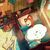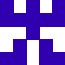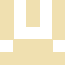# mui.back()重写之后，有显示再按一次退出，但程序先退出了，我监控返回键也是这样。找不到Bug，求大神支招。

``````    // H5 plus事件处理
ws = plus.webview.currentWebview();
wo = ws.opener();
ws.show("slide-in-right", 300);
window.setTimeout(function() {
var s = ws.getStyle();
s.left = "0%";
ws.setStyle(s);
}, 100);

//关闭当前页外的所有页面
var all = plus.webview.all();
var current = plus.webview.currentWebview().id;
for(var i=0,len=all.length;i<len;i++){
if(all[i].id!==current){
all[i].close();
}
}

//首页返回键处理(有效果，但程序先退出了)
//处理逻辑：1秒内，连续两次按返回键，则退出应用；
var first = null;
//首次按键，提示‘再按一次退出应用’
if (!first) {
first = new Date().getTime();
mui.toast('再按一次退出应用');
setTimeout(function() {
first = null;
}, 1000);
} else {
if (new Date().getTime() - first < 1000) {
plus.runtime.quit();
}
}
}, false);
});

}
if(window.plus) {
} else {
}``````
2016-08-23 10:41 负责人:无 分享wenju - https://www.mescroll.com -- 精致的下拉刷新和上拉加载组件

``````//首页返回键处理,处理逻辑：1秒内,连续两次按返回键，则退出应用
var first = null;
mui.back = function() {
if (!first) {//首次按键，提示‘再按一次退出应用’
first = (new Date()).getTime();
mui.toast('再按一次退出应用');
setTimeout(function() {
first = null;
}, 1000);
} else {
if ((new Date()).getTime() - first < 1000) {
plus.runtime.quit();
}
}
};``````wenju - https://www.mescroll.com -- 精致的下拉刷新和上拉加载组件``````mui.init();
var first = null;
mui.back = function() {
if (!first) {//首次按键，提示‘再按一次退出应用’
first = (new Date()).getTime();
mui.toast('再按一次退出应用11111111');
console.log("qweqweqwewqd");
setTimeout(function() {
first = null;
}, 1000);
} else {
console.log("asdasdasd");
if ((new Date()).getTime() - first < 1000) {
plus.runtime.quit();
}
}
};
var Intent;
var firstPress = null;
switch(plus.os.name) {
case "Android":
Intent = plus.android.importClass("android.content.Intent");
break;
case "iOS":
Intent = plus.ios.importClass("GKLocalPlayer");
break;
default:
// 其它平台
break;
}

//          if(!firstPress) {
//              firstPress = new Date().getTime();
//              mui.toast('再按一次退出应用');
//              setTimeout(function() {
//                  firstPress = null;
//              }, 2000);
//          } else {
//              mui.toast('又按了一次');
//              if(new Date().getTime() - firstPress < 2000) {
//                  plus.runtime.quit();
//              }
//          }
//      }, false);
//      var viewModel = new LP_ViewModel();
//      ko.applyBindings(viewModel);
}
if(window.plus) {
} else {
}
});``````mui.back = function() {
var data = 0;
mui.back = function() {
var first = null;
if (!first) {//首次按键，提示‘再按一次退出应用’
first = 1;
mui.toast('再按一次退出应用');
//console.log(data);
data += 1;
setTimeout(function() {
first = null;
data = 0;
}, 1000);
}
if(data == 3) {
// console.log(data);
plus.runtime.quit();
}
};
};``````mui.init({